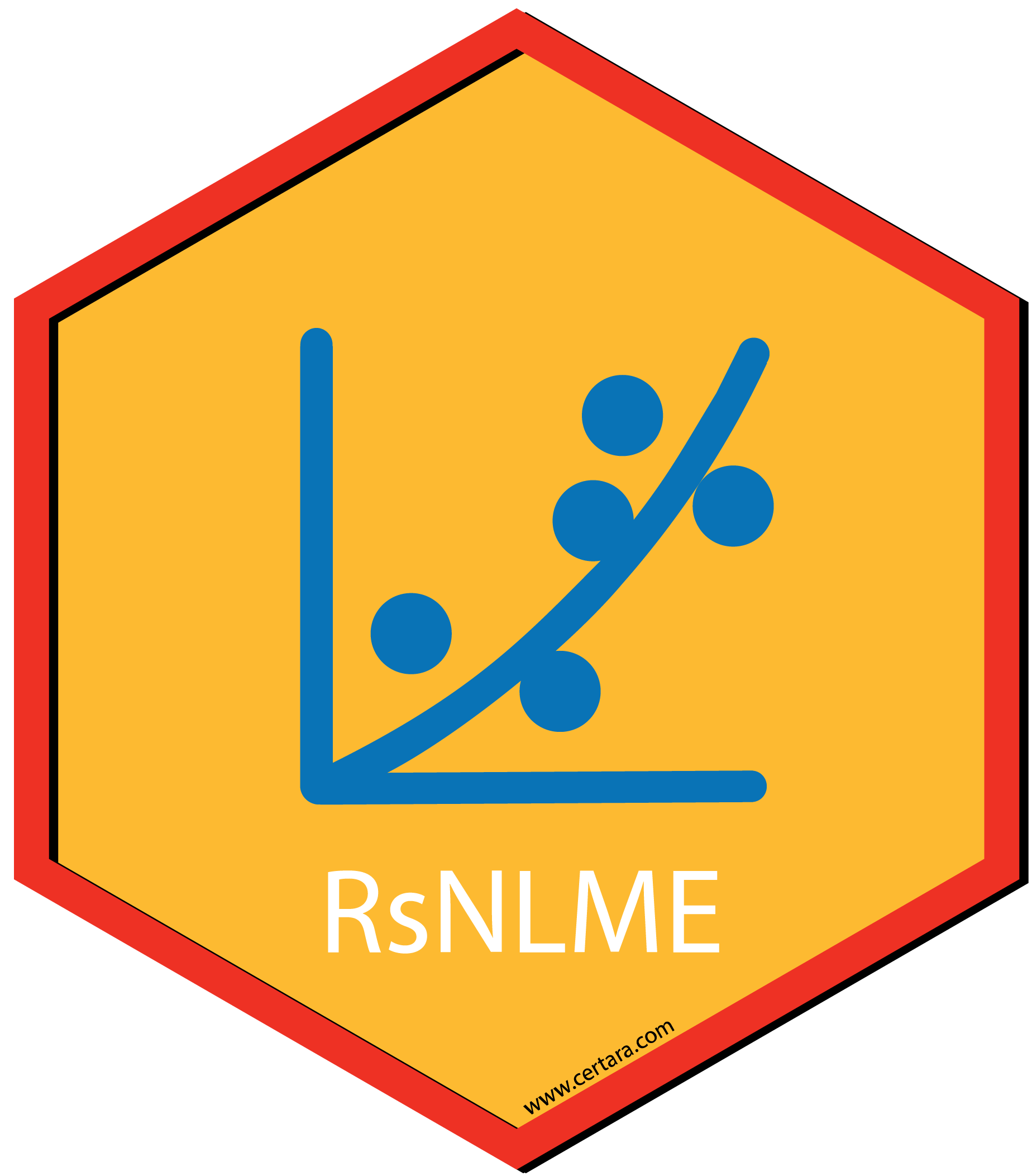## Overview

The purpose of this vignette is to demonstrate how to:

• Fit a model defined by PML codes, where
• the PK portion of the model is described by a one-compartment model with first-order absorption
• the PD portion of the model is described by an Emax model and a categorical model with three categories
• Import estimation results to xpose database to create some commonly used diagnostic plots for each continuous observed variable
• Perform VPC for the model
• Create VPC plots through open source package tidyvpc (command-line usage) and VPC results Shiny app (in Certara.VPCResults package)

We assume that all the necessary packages are loaded and the directory with NLME Executables is given as an environment variable (INSTALLDIR).

  # loading the package
library(Certara.RsNLME)
library(data.table)
library(dplyr)
library(xpose)
library(Certara.Xpose.NLME)
library(ggplot2)
library(Certara.ModelResults)
library(Certara.VPCResults)
library(tidyvpc)

# Check the environment variable
Sys.getenv("INSTALLDIR")
 "C:\\Program Files\\Certara\\NLME_Engine"

## Create the textual model

We will use the data OneCpt1stOrderAbsorp_Emax_CategoricalModel.csv and the PML model file OneCpt1stOrderAbsorp_Emax_CategoricalModel.mdl distributed with the Certara.RsNLME package. First, we will import the data

  filename <- system.file("vignettesdata/OneCpt1stOrderAbsorp_Emax_CategoricalModel.csv",
package = "Certara.RsNLME",
mustWork = TRUE)
dt_InputDataSet <- fread(filename)

Next we will locate the PML model file and then create a textual model object for it.


filename <- system.file("vignettesdata/OneCpt1stOrderAbsorp_Emax_CategoricalModel.mdl",
package = "Certara.RsNLME",
mustWork = TRUE)

# Load the PML codes and link it to associated input data to create a model object
model <- textualmodel(modelName = "OneCpt1stOrderAbsorp_Emax_CategoricalModel",
mdl = filename,
data = dt_InputDataSet)

Let’s view the model and its associated column mappings, and then map those un-mapped model variables to their corresponding input data columns:

  # View the model and its associated column mappings
print(model)

Model Overview
-------------------------------------------
Model Name        :  OneCpt1stOrderAbsorp_Emax_CategoricalModel
Working Directory :  C:/Users/jcraig/Documents/GitHub/R-RsNLME/vignettes/OneCpt1stOrderAbsorp_Emax_CategoricalModel
Model Type        :  Textual

PML
-------------------------------------------
test(){

# ===============================================================
# PK model: one compartment model with 1st order absorption
# ===============================================================
cfMicro(A1, Cl / V, first = (Aa = Ka))
dosepoint(Aa)
C = A1 / V

# residual error model
error(CEps = 0.1)
observe(CObs = C * (1 + CEps))

# ----------------------------------------------------------------
# PK model parameters
# ----------------------------------------------------------------
# Structural model parameters
stparm(Ka = exp(tvlogKa + nlogKa))
stparm(V = exp(tvlogV + nlogV))
stparm(Cl = exp(tvlogCl + nlogCl))

# fixed effects
fixef(tvlogKa = c(, -1, ))
fixef(tvlogV = c(, 2, ))
fixef(tvlogCl = c(, 0, ))

# random effects
ranef(diag(nlogV, nlogCl, nlogKa) = c(1, 1, 1))

# ================================================================
# PD model
# ================================================================
E = Emax * C / (EC50 + C)

## Residual error model
error(EEps = 0.1)
observe(EObs = E * (1 + EEps))

## Categorical model
multi(CategoricalObs, ilogit, -E, -(E + CatParam))

# ----------------------------------------------------------------
# Categorical model parameters
# ----------------------------------------------------------------
# structural model parameters
stparm(EC50 = exp(tvlogEC50 + nlogEC50))
stparm(Emax = exp(tvlogEmax + nlogEmax))
stparm(CatParam = exp(tvlogCatParam + nlogCatParam))

# fixed effects
fixef(tvlogEC50 = c(, 2, ))
fixef(tvlogEmax = c(, -2, ))
fixef(tvlogCatParam = c(, 1, ))

# random effects
ranef(diag(nlogEC50, nlogEmax, nlogCatParam) = c(1, 1, 1))
}

Structural Parameters
-------------------------------------------
Ka V Cl EC50 Emax CatParam
-------------------------------------------
Column Mappings
-------------------------------------------
Model Variable Name : Data Column name
id                  : ?
time                : time
Aa                  : ?
CObs                : CObs
EObs                : EObs
CategoricalObs      : CategoricalObs

# Manually map those un-mapped model variables to their corresponding input data columns
model <- model %>%
colMapping(c(id = "SubID", Aa = "dose_Aa"))


## Model Fitting

Next, we will run the model using the fitmodel function with default host. We will use the QRPEM method for fitting. We will also output residuals PCWRES with the number of replicates set to be 1000 (Note: PCWRES is not outputted by default).

  job <- fitmodel(model, method = "QRPEM", numRepPCWRES = 1000)
Using MPI host with 4 cores
sharedDirectory is not given in the host class instance.
Valid NLME_ROOT_DIRECTORY is not given, using current working directory:
C:/Users/jcraig/Documents/GitHub/R-RsNLME/vignettes
  print(job$Overall) Scenario RetCode LogLik -2LL AIC BIC nParm nObs nSub 1: WorkFlow 1 2711.294 -5422.588 -5394.588 -5297.671 14 7500 300 EpsShrinkage Condition 1: 0.09678 3.46327 ## Diagnostic Plots We will use the xposeNlme function from the Certara.Xpose.NLME package to import estimation results to xpose database to create some commonly used diagnostic plots. All the functions provided in the xpose package can be used. Here we only demonstrate several of these functions.  ## Imports results of an NLME run into xpose database to create commonly used diagnostic plots xp <- xposeNlme(dir = model@modelInfo@workingDir, modelName = "OneCpt1stOrderAbsorp_Emax_CategoricalModel") ## Filter out CategoricalObs xp <- xp %>% filter(ObsName != "CategoricalObs") ## observations against population predictions dv_vs_pred(xp, type = "p", facets = "ObsName", subtitle = "-2LL: @ofv")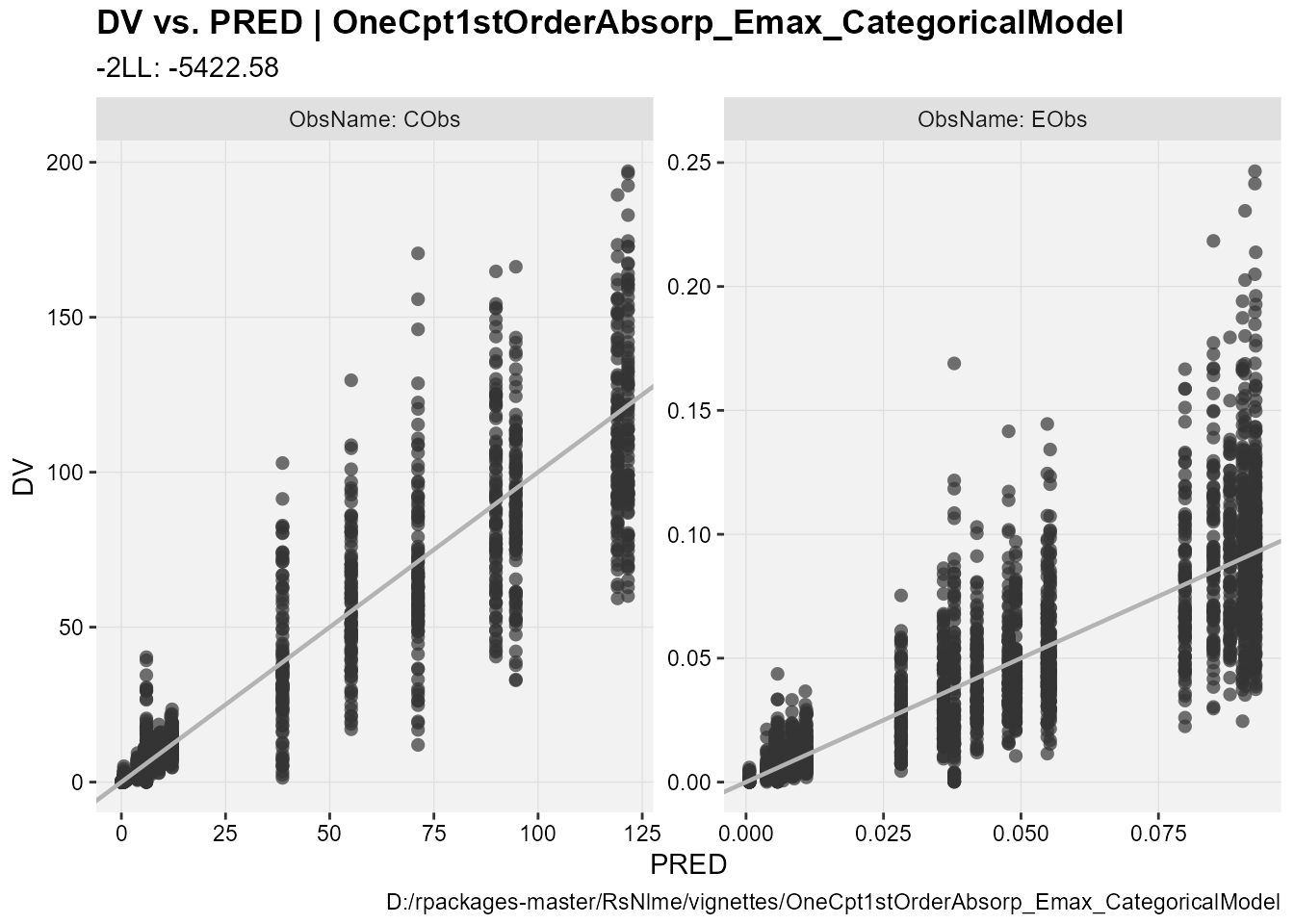## observations against individual predictions dv_vs_ipred(xp, type = "p", facets = "ObsName", subtitle = "-2LL: @ofv, Eps shrinkage: @epsshk")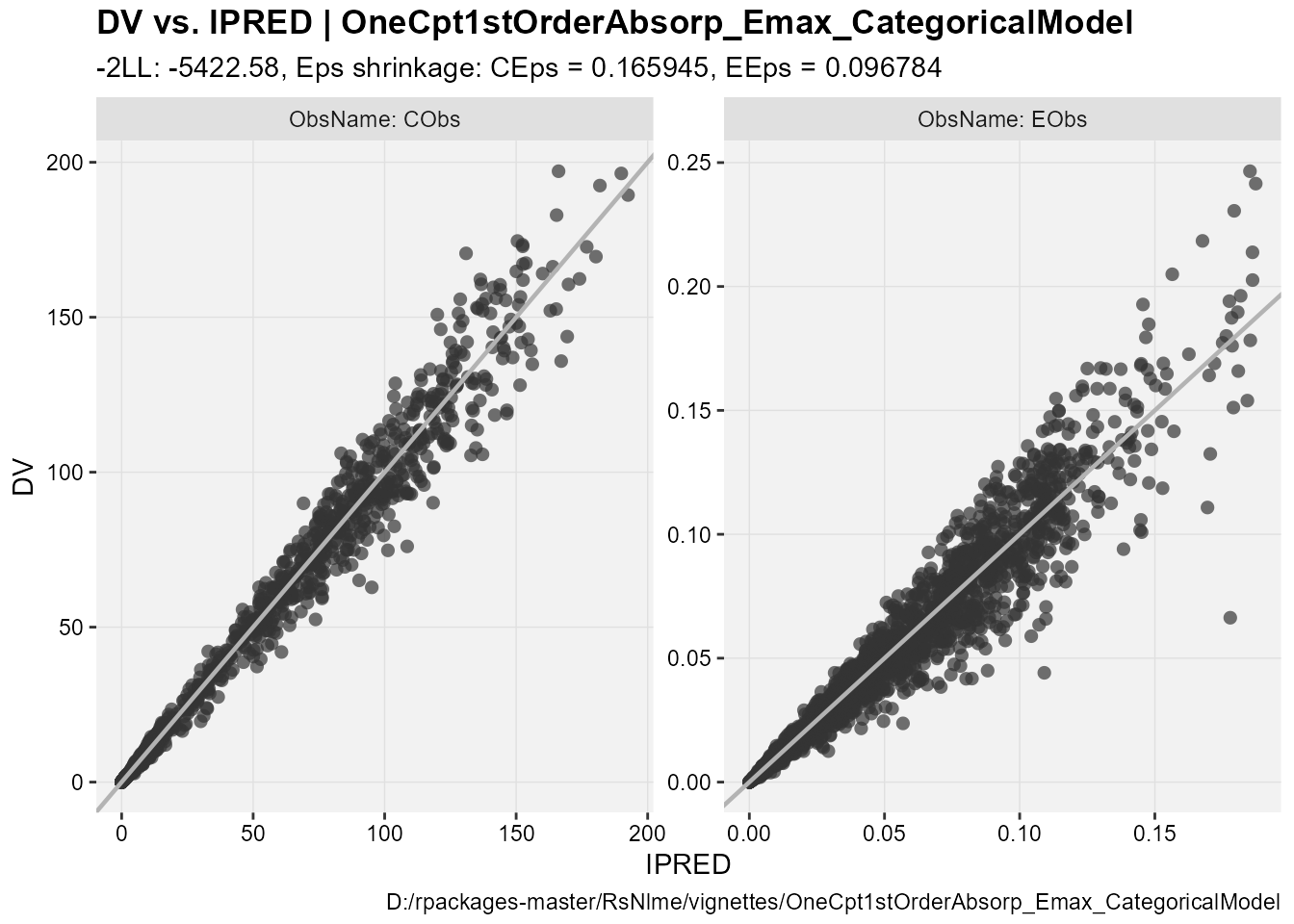## CWRES against population predictions res_vs_pred(xp, res = "CWRES", type = "ps", facets = "ObsName", subtitle = "-2LL: @ofv") geom_smooth() using formula 'y ~ x' geom_smooth() using formula 'y ~ x'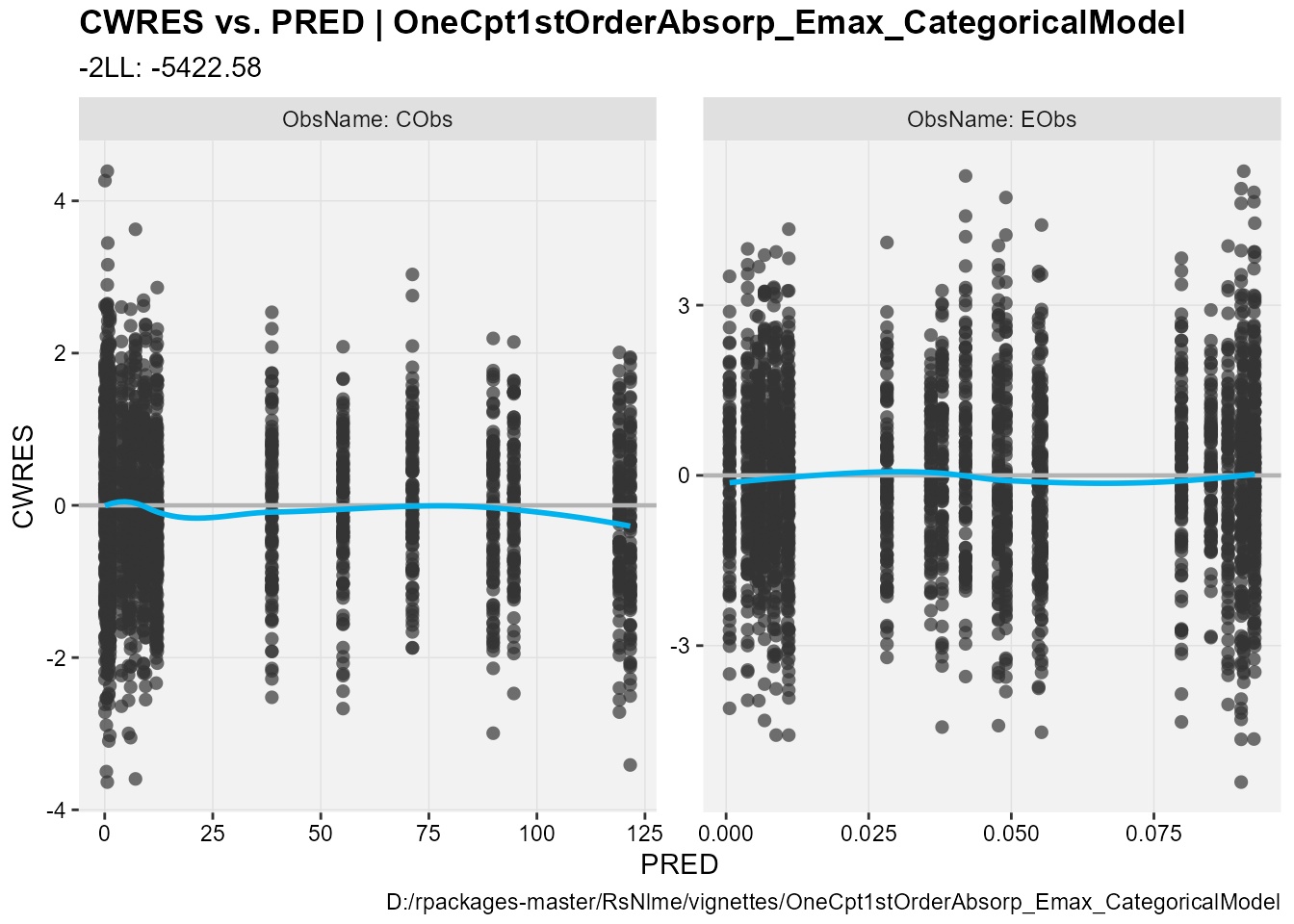## CWRES against the independent variable res_vs_idv(xp, res = "CWRES", type = "ps", facets = "ObsName", subtitle = "-2LL: @ofv") geom_smooth() using formula 'y ~ x' geom_smooth() using formula 'y ~ x'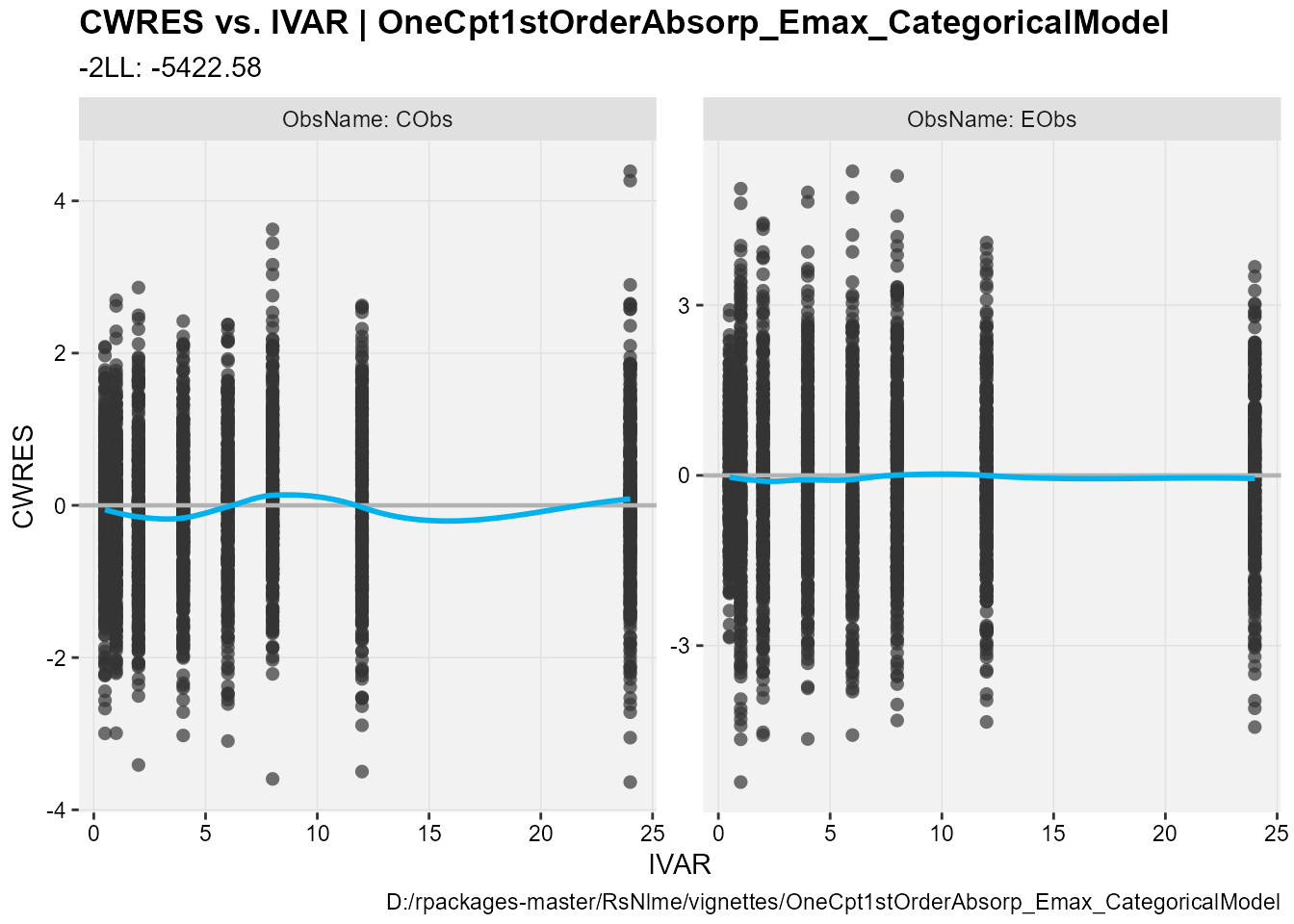## PCWRES against population predictions res_vs_pred(xp, res = "PCWRES", type = "ps", facets = "ObsName", subtitle = "-2LL: @ofv") geom_smooth() using formula 'y ~ x' geom_smooth() using formula 'y ~ x'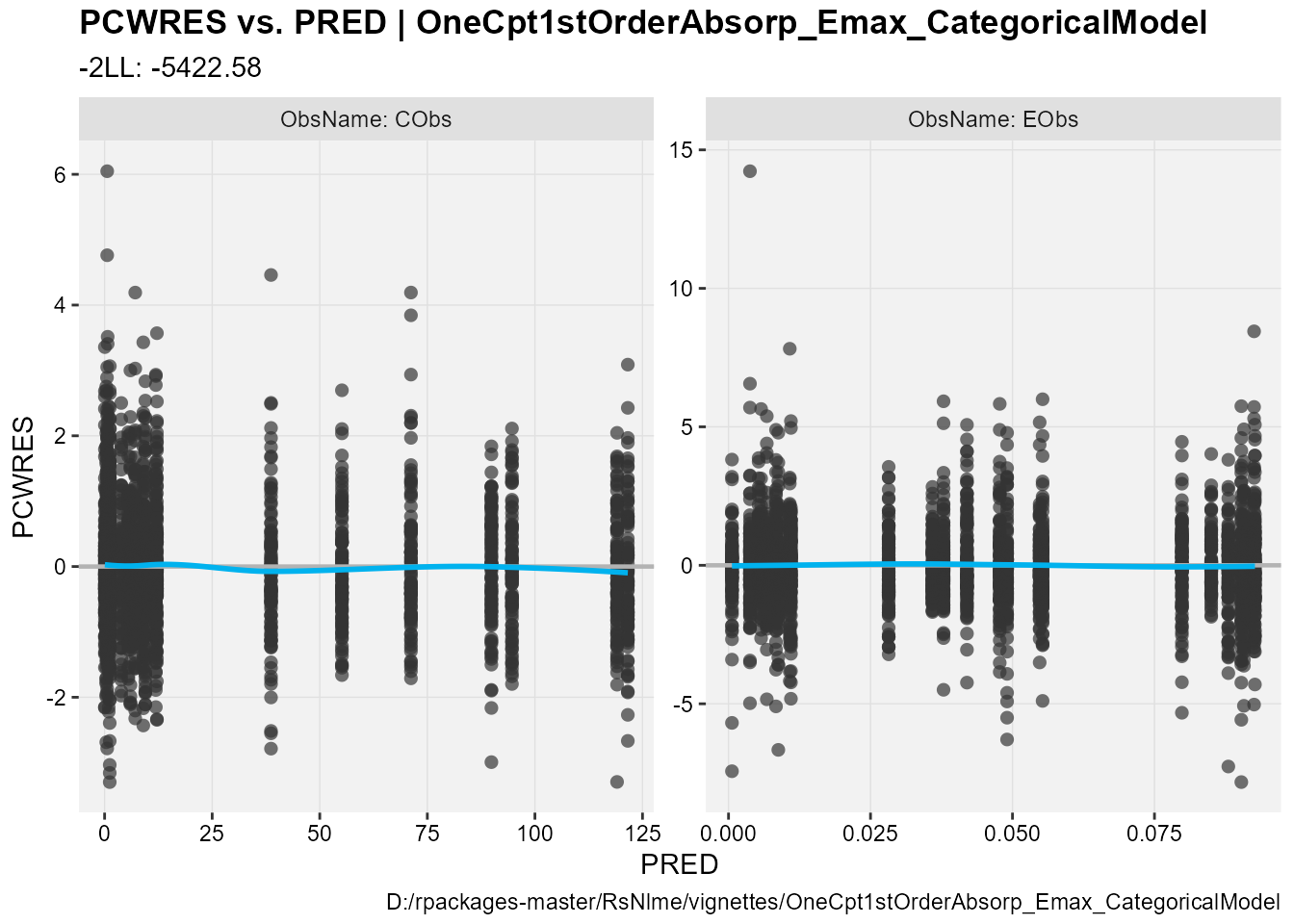## PCWRES against the independent variable res_vs_idv(xp, res = "PCWRES", type = "ps", facets = "ObsName", subtitle = "-2LL: @ofv") geom_smooth() using formula 'y ~ x' geom_smooth() using formula 'y ~ x'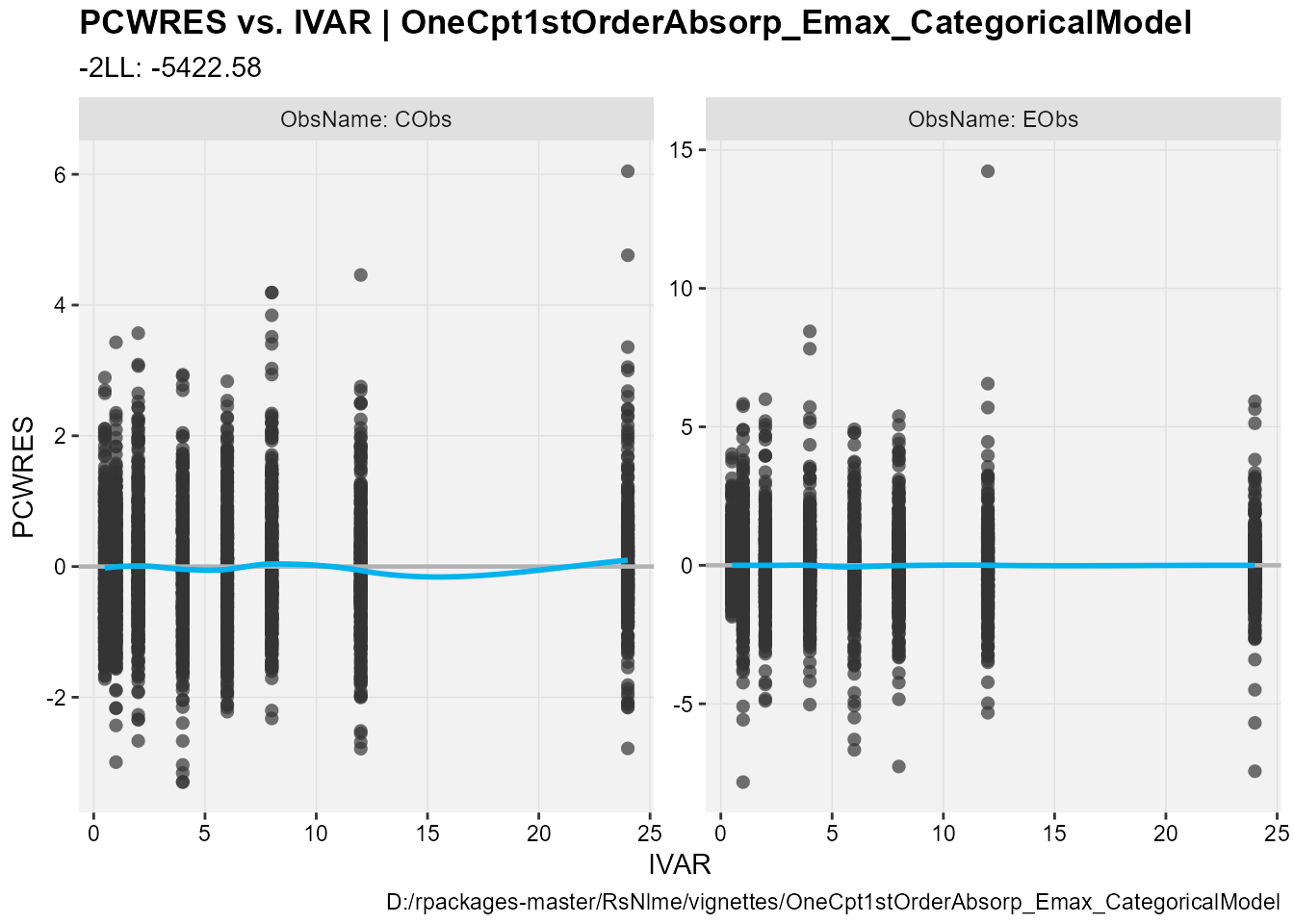Alternatively, one can view/customize diagnostic plots as well as estimation results using the Certara.ModelResults Shiny application, which can also be used to generate .R and/or .Rmd code based on operations performed in the GUI. For installation and usage details, please visit the following link. Here we only demonstrate how to invoke this Shiny app through either the NlmePmlModel object or the xpose_data object created above. library(Certara.ModelResults) ## Invoke model results shiny app through model object defined above resultsUI(model = model) ## Alternatively, one can invoke model results shiny app through xpose data object created above resultsUI(xpdb = xp) ## VPC We will use the copyModel function to copy the model into a new object and accept final parameter estimates from fitting run as initial estimates for VPC simulation:  modelVPC <- copyModel(model, acceptAllEffects = TRUE, modelName = "OneCpt1stOrderAbsorp_Emax_CategoricalModel_VPC") ## View model print(modelVPC) Model Overview ------------------------------------------- Model Name : OneCpt1stOrderAbsorp_Emax_CategoricalModel_VPC Working Directory : C:/Users/jcraig/Documents/GitHub/R-RsNLME/vignettes/OneCpt1stOrderAbsorp_Emax_CategoricalModel_VPC Model Type : Textual PML ------------------------------------------- test(){ # =============================================================== # PK model: one compartment model with 1st order absorption # =============================================================== cfMicro(A1, Cl / V, first = (Aa = Ka)) dosepoint(Aa) C = A1 / V # residual error model error(CEps = 0.121544989414485) observe(CObs = C * (1 + CEps)) # ---------------------------------------------------------------- # PK model parameters # ---------------------------------------------------------------- # Structural model parameters stparm(Ka = exp(tvlogKa + nlogKa)) stparm(V = exp(tvlogV + nlogV)) stparm(Cl = exp(tvlogCl + nlogCl)) # fixed effects fixef(tvlogKa = c(,-0.356862376082258,)) fixef(tvlogV = c(,1.63701201337159,)) fixef(tvlogCl = c(,-0.224407387640359,)) # random effects ranef(diag(nlogV, nlogCl, nlogKa) = c(0.086623473, 0.1052053, 0.11503702)) # ================================================================ # PD model # ================================================================ E = Emax * C / (EC50 + C) ## Residual error model error(EEps = 0.179381190657918) observe(EObs = E * (1 + EEps)) ## Categorical model multi(CategoricalObs, ilogit, -E, -(E + CatParam)) # ---------------------------------------------------------------- # Categorical model parameters # ---------------------------------------------------------------- # structural model parameters stparm(EC50 = exp(tvlogEC50 + nlogEC50)) stparm(Emax = exp(tvlogEmax + nlogEmax)) stparm(CatParam = exp(tvlogCatParam + nlogCatParam)) # fixed effects fixef(tvlogEC50 = c(,2.2899579614783,)) fixef(tvlogEmax = c(,-2.30045875119669,)) fixef(tvlogCatParam = c(,0.418715524594699,)) # random effects ranef(diag(nlogEC50, nlogEmax, nlogCatParam) = c(0.066093482, 0.10266479, 0.15212821)) } Structural Parameters ------------------------------------------- Ka V Cl EC50 Emax CatParam ------------------------------------------- Column Mappings ------------------------------------------- Model Variable Name : Data Column name id : SubID time : time Aa : dose_Aa CObs : CObs EObs : EObs CategoricalObs : CategoricalObs Now, let’s run VPC using the vpcmodel function with the default host, default values for the relevant NLME engine arguments, and PRED outputted. Note: Here we define VPC argument, outputPRED, through ellipsis (additional argument). Alternatively, one can define VPC arguments through vpcParams argument.  vpcJob <- vpcmodel(modelVPC, outputPRED = TRUE) Using localhost without parallelization. NLME Job sharedDirectory is not given in the host class instance. Valid NLME_ROOT_DIRECTORY is not given, using current working directory: C:/Users/jcraig/Documents/GitHub/R-RsNLME/vignettes Compiling 1 of 1 NLME models The model compiled Trying to generate job results... Copying tables predcheck_bql.csv, predcheck0.csv, predcheck0_cat.csv, predcheck1.csv, predcheck1_cat.csv, predcheck2.csv, predcheck2_cat.csv, predout.csv Finished summarizing results. Transferring data and loading the results... Done generating job results. VPC/Simulation results are ready in C:/Users/jcraig/Documents/GitHub/R-RsNLME/vignettes/OneCpt1stOrderAbsorp_Emax_CategoricalModel_VPC Loading the results Loading predcheck_bql.csv Loading predcheck0.csv Loading predcheck0_cat.csv Loading predcheck1.csv Loading predcheck1_cat.csv Loading predcheck2.csv Loading predcheck2_cat.csv Loading predout.csv ## predcheck0 contains observed data for all continuous observed variables dt_ObsData_ContinuousObs <- vpcJob$predcheck0

## predcheck0_cat contains observed data for Categorical/count observed variables
dt_ObsData_CategoricalObs <- vpcJob$predcheck0_cat ## predout contains simulated data for all observed variables dt_SimData <- vpcJob$predout

Next we will create VPC plots through tidyvpc package. The tidyvpc package provides support for both continuous and categorical VPC using both binning and binless methods. For details on this package, please visit the following link. Note that this example contains 3 observed variable with PRED outputted. Hence, to use this package, we have to do some data preprocessing on both simulated and observed data to meet the requirements set by the tidyvpc package.

First we will process the simulated data output to pass to the simulated() function in the tidyvpc package, creating a separate data.frame for each of our DV:

  ## Extract simulated data for observed variable "CObs"
dt_SimData_tidyvpc_CObs <- dt_SimData[OBSNAME == "CObs"]
## Extract simulated data for observed variable "EObs"
dt_SimData_tidyvpc_EObs <- dt_SimData[OBSNAME == "EObs"]
## Extract simulated data for observed variable "CategoricalObs"
dt_SimData_tidyvpc_CategoricalObs <- dt_SimData[OBSNAME == "CategoricalObs"]

Next, we will process the observed data output to pass to the observed() function in the tidyvpc package, creating a separate data.frame for each of our DV as we did for the simulated data output:

  ## Extract observed data for observed variable "CObs"
dt_ObsData_ContinuousObs_tidyvpc_CObs <- dt_ObsData_ContinuousObs[ObsName == "CObs"]
## Extract observed data for observed variable "EObs"
dt_ObsData_ContinuousObs_tidyvpc_EObs <- dt_ObsData_ContinuousObs[ObsName == "EObs"]
## Extract observed data for observed variable "CategoricalObs"
dt_ObsData_CategoricalObs_tidyvpc <- dt_ObsData_CategoricalObs[ObsName == "CategoricalObs"]

Finally, we will add the $PRED column from REPLICATE == 0 ($PRED may be extracted from any REPLICATE) in the simulated data to our observed data in order to perform a prediction-corrected VPC:

  ## Add PRED from REPLICATE == 0 of simulated data (CObs) to observed data (CObs)
dt_ObsData_ContinuousObs_tidyvpc_CObs$PRED <- as.numeric(dt_SimData_tidyvpc_CObs[REPLICATE == 0]$PRED)

## Add PRED from REPLICATE == 0 of simulated data (EObs) to observed data (EObs)
dt_ObsData_ContinuousObs_tidyvpc_EObs$PRED <- as.numeric(dt_SimData_tidyvpc_EObs[REPLICATE == 0]$PRED)

Now we can create VPC plots.

  ### Create a binless VPC plot for CObs

binless_VPC_CObs <- observed(dt_ObsData_ContinuousObs_tidyvpc_CObs, x = IVAR, yobs = DV) %>%
simulated(dt_SimData_tidyvpc_CObs, ysim = DV) %>%
binless() %>%
vpcstats()
plot(binless_VPC_CObs)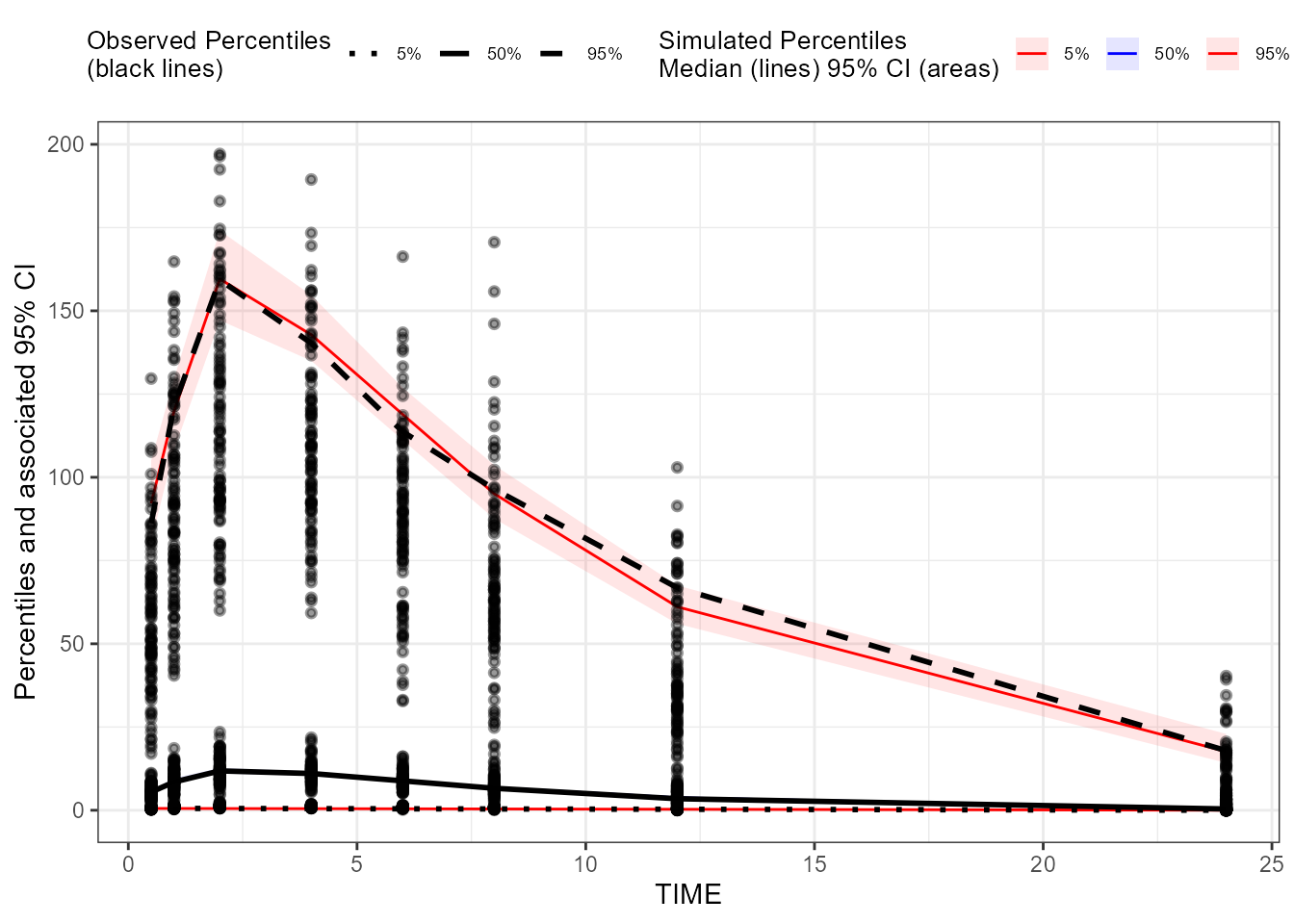### Create a binless pcVPC plot for CObs
binless_pcVPC_CObs <- observed(dt_ObsData_ContinuousObs_tidyvpc_CObs,
x = IVAR,
yobs = DV) %>%
simulated(dt_SimData_tidyvpc_CObs, ysim = DV) %>%
binless(loess.ypc = TRUE) %>%
predcorrect(pred = PRED) %>%
vpcstats()
plot(binless_pcVPC_CObs)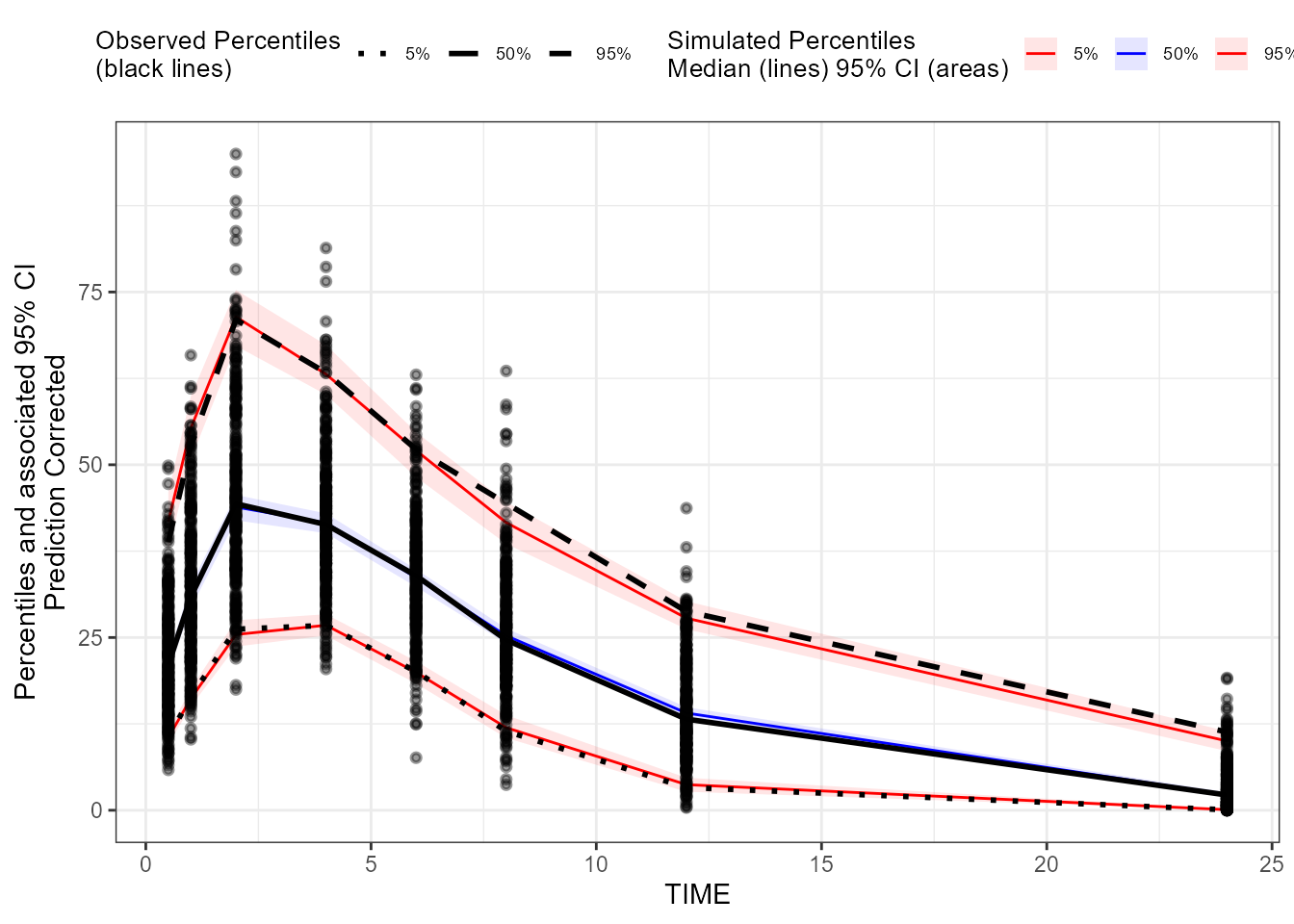### Create a binless VPC plot for EObs
binless_VPC_EObs <- observed(dt_ObsData_ContinuousObs_tidyvpc_EObs, x = IVAR, yobs = DV) %>%
simulated(dt_SimData_tidyvpc_EObs, ysim = DV) %>%
binless() %>%
vpcstats()
plot(binless_VPC_EObs)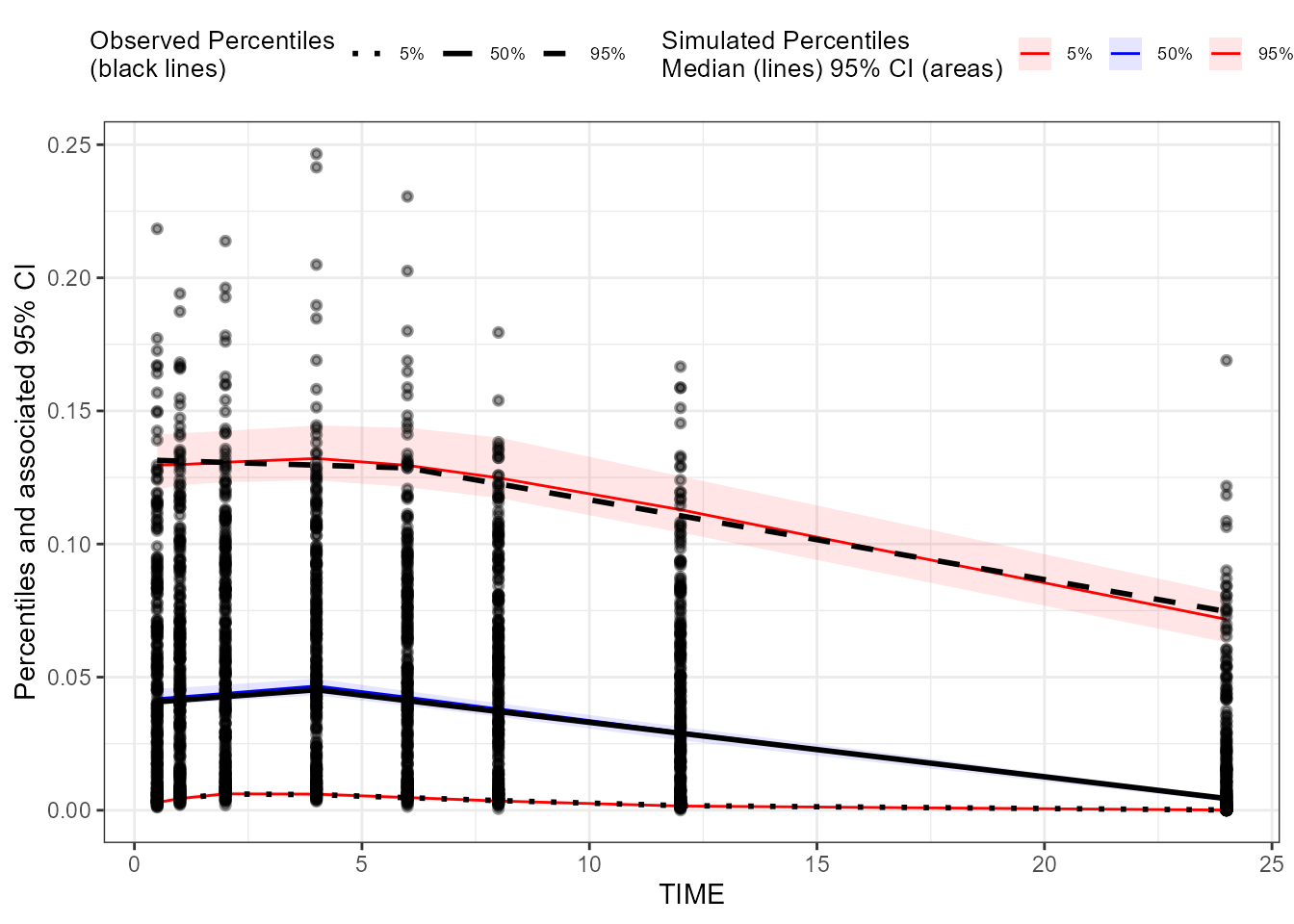### Create a binless pcVPC plot for EObs
binless_pcVPC_EObs <- observed(dt_ObsData_ContinuousObs_tidyvpc_EObs, x = IVAR, yobs = DV) %>%
simulated(dt_SimData_tidyvpc_EObs, ysim = DV) %>%
binless(loess.ypc = TRUE) %>%
predcorrect(pred = PRED) %>%
vpcstats()
plot(binless_pcVPC_EObs)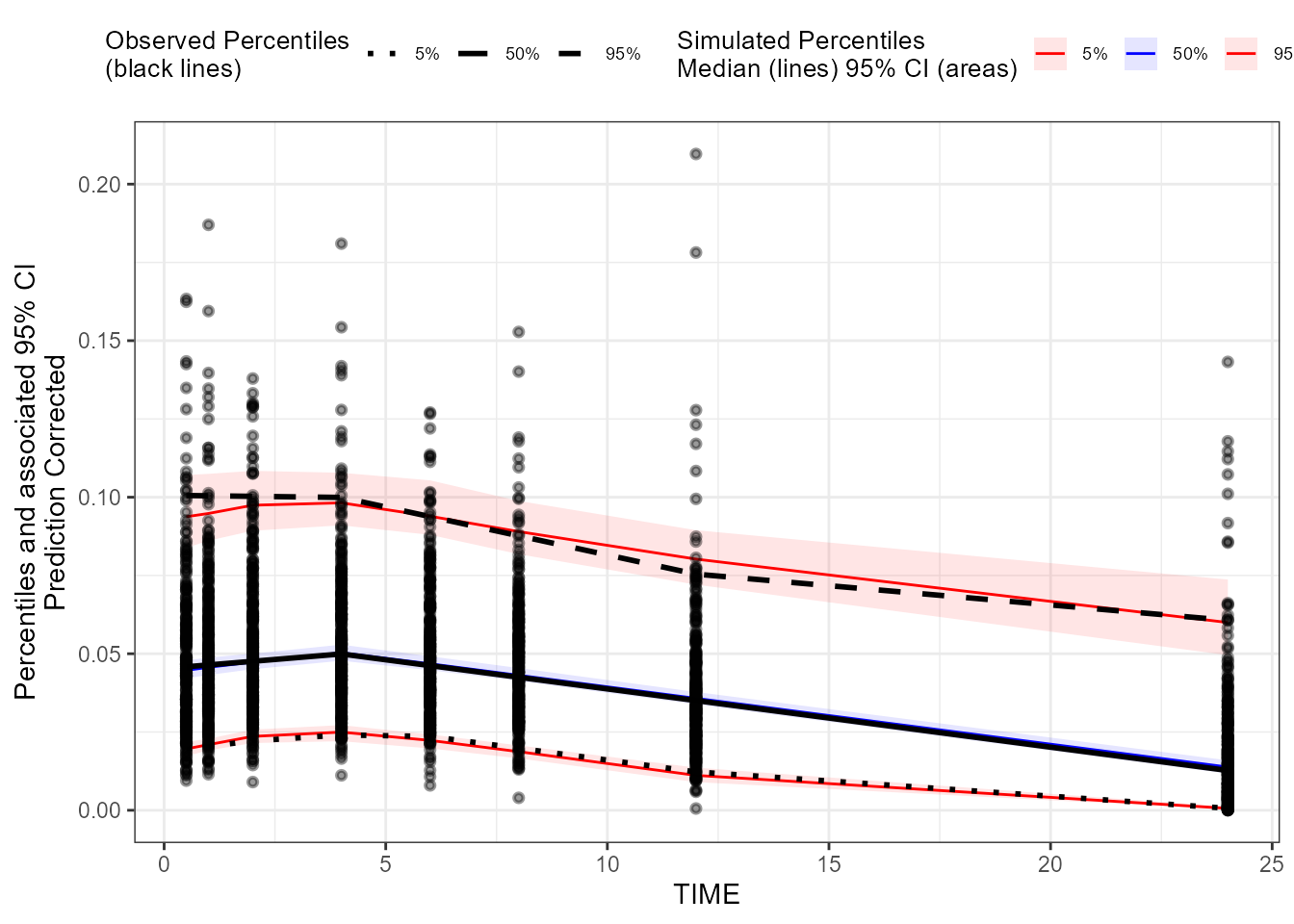### Create a binless VPC plot for CatgoricalObs
binless_VPC_CategoricalObs <- observed(dt_ObsData_CategoricalObs_tidyvpc,
x = IVAR,
yobs = DV) %>%
simulated(dt_SimData_tidyvpc_CategoricalObs, ysim = DV) %>%
binless() %>%
vpcstats(vpc.type = "categorical")

plot(binless_VPC_CategoricalObs
, facet = TRUE
, facet.scales = "fixed"
, legend.position = "bottom"
)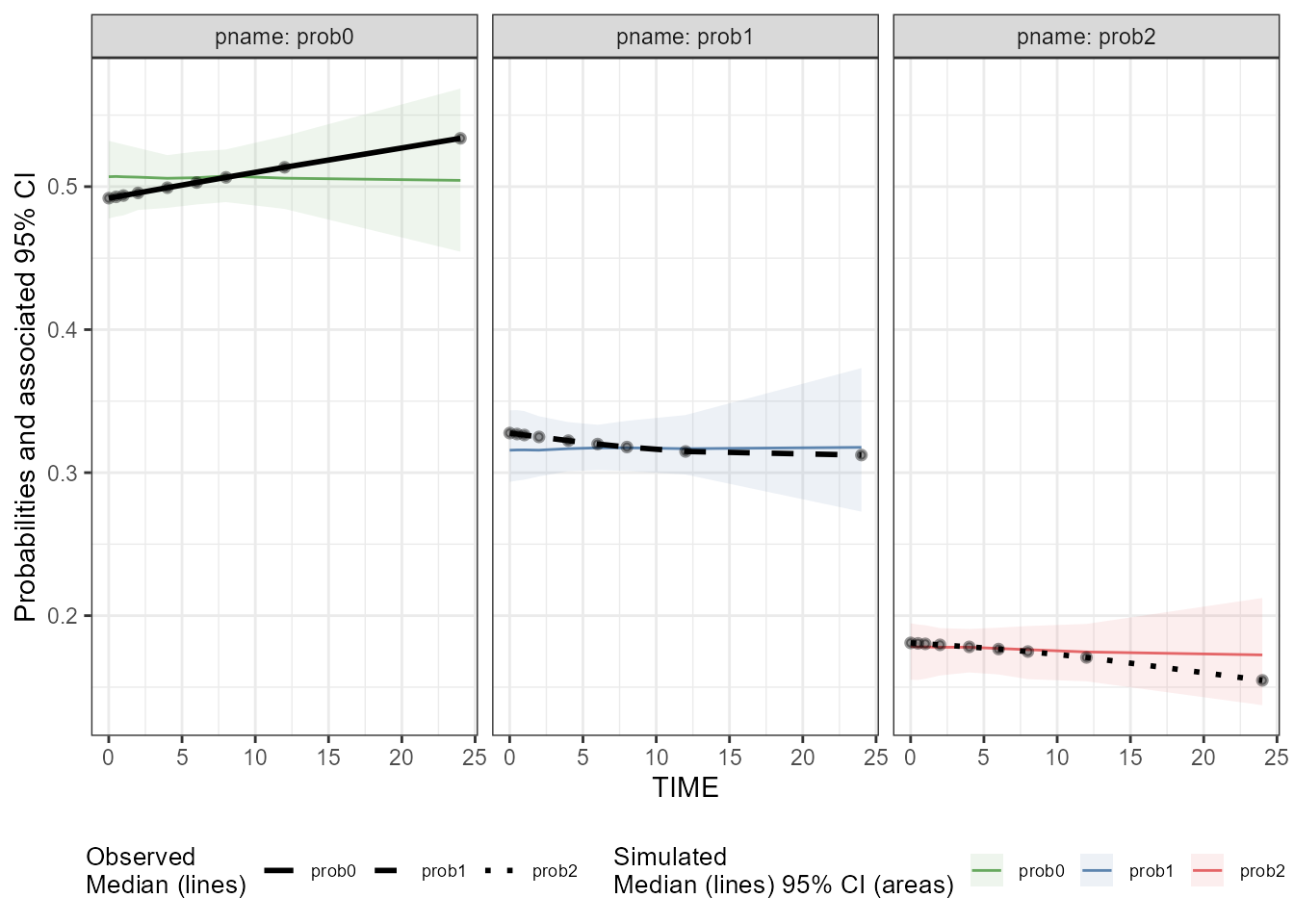### Create a binning VPC plot for CObs: binning on x-variable itself
binning_VPC_CObs <- observed(dt_ObsData_ContinuousObs_tidyvpc_CObs, x = IVAR, yobs = DV) %>%
simulated(dt_SimData_tidyvpc_CObs, ysim = DV) %>%
binning(bin = IVAR) %>%
vpcstats()
plot(binning_VPC_CObs)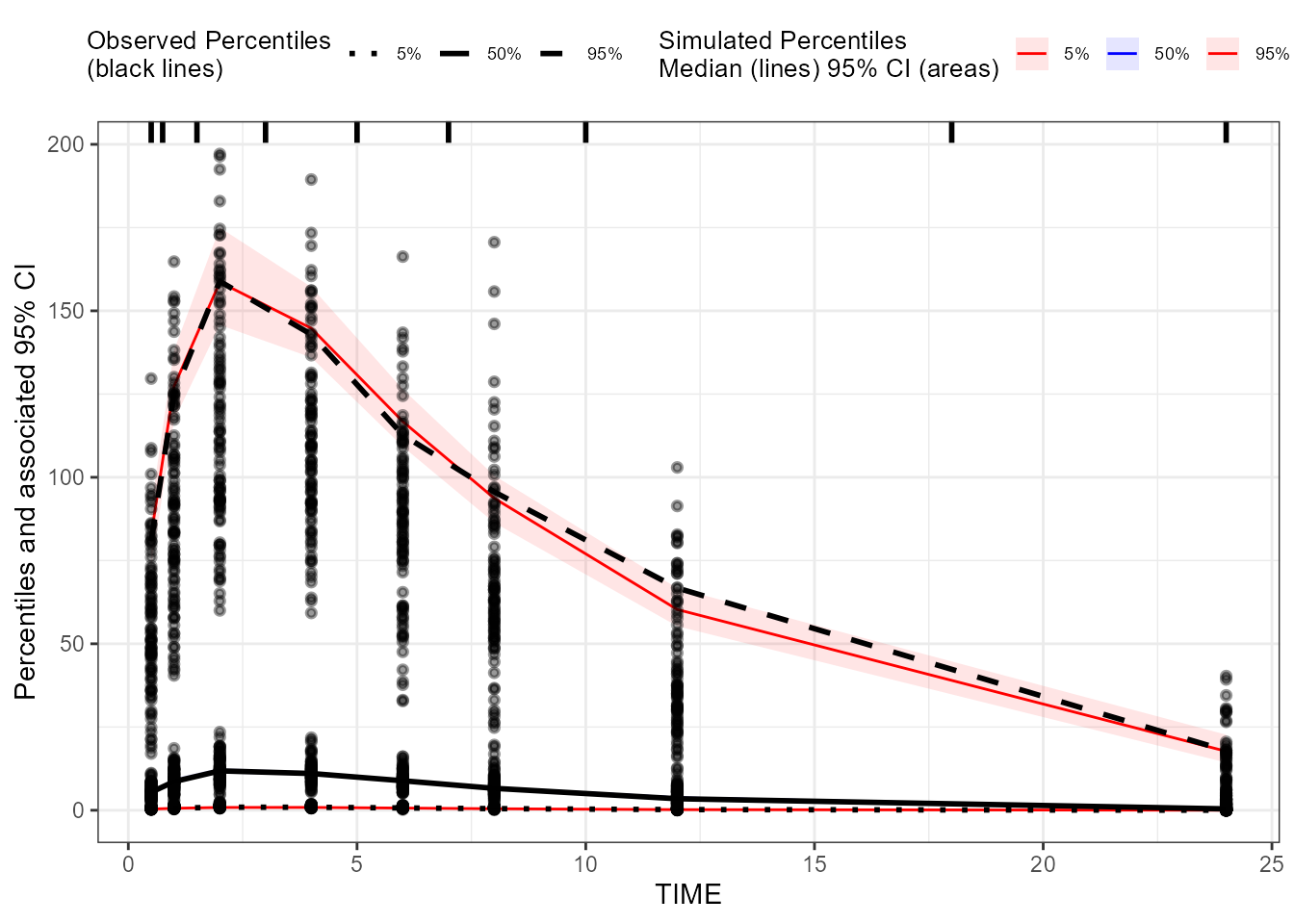### Create a binning pcVPC plot for CObs: binning on x-variable itself
binning_pcVPC_CObs <- observed(dt_ObsData_ContinuousObs_tidyvpc_CObs,
x = IVAR,
yobs = DV) %>%
simulated(dt_SimData_tidyvpc_CObs, ysim = DV) %>%
binning(bin = IVAR) %>%
predcorrect(pred = PRED) %>%
vpcstats()
plot(binning_pcVPC_CObs)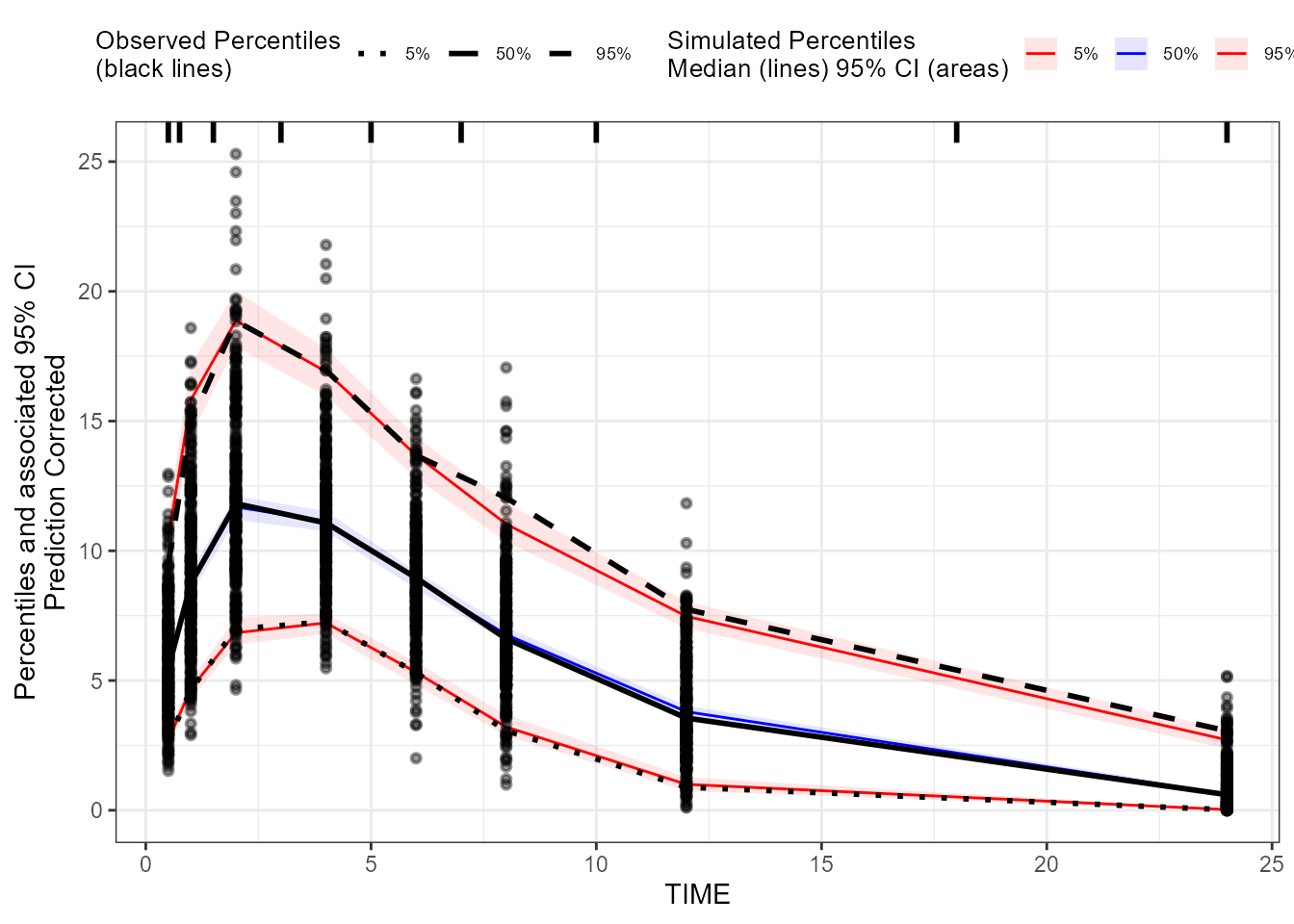### Create a binning VPC plot for EObs: binning on x-variable itself
binning_VPC_EObs <- observed(dt_ObsData_ContinuousObs_tidyvpc_EObs, x = IVAR, yobs = DV) %>%
simulated(dt_SimData_tidyvpc_EObs, ysim = DV) %>%
binning(bin = IVAR) %>%
vpcstats()
plot(binning_VPC_EObs)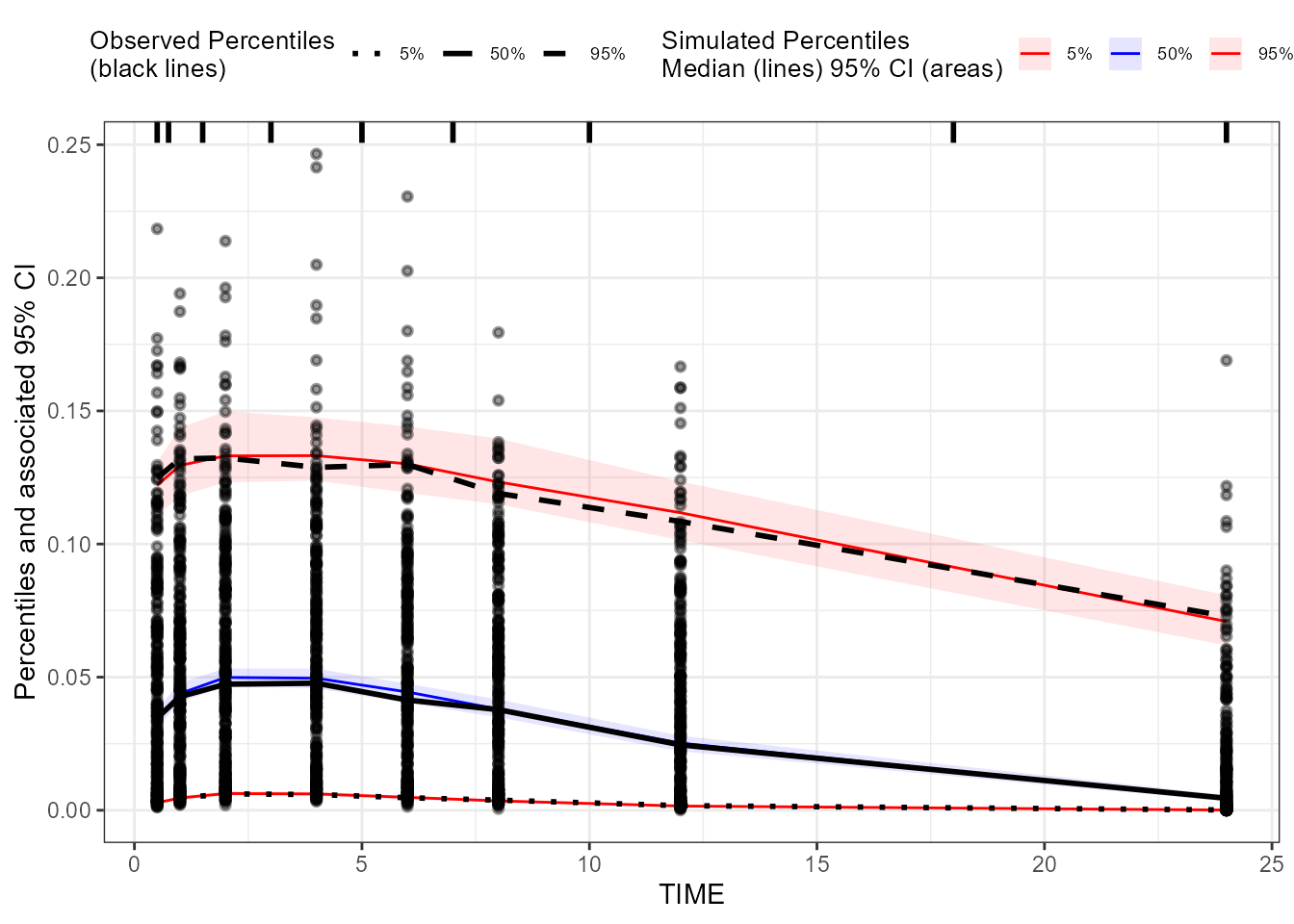### Create a binning pcVPC plot for EObs: binning on x-variable itself
binning_pcVPC_EObs <- observed(dt_ObsData_ContinuousObs_tidyvpc_EObs, x = IVAR, yobs = DV) %>%
simulated(dt_SimData_tidyvpc_EObs, ysim = DV) %>%
binning(bin = IVAR) %>%
predcorrect(pred = PRED) %>%
vpcstats()
plot(binning_pcVPC_EObs)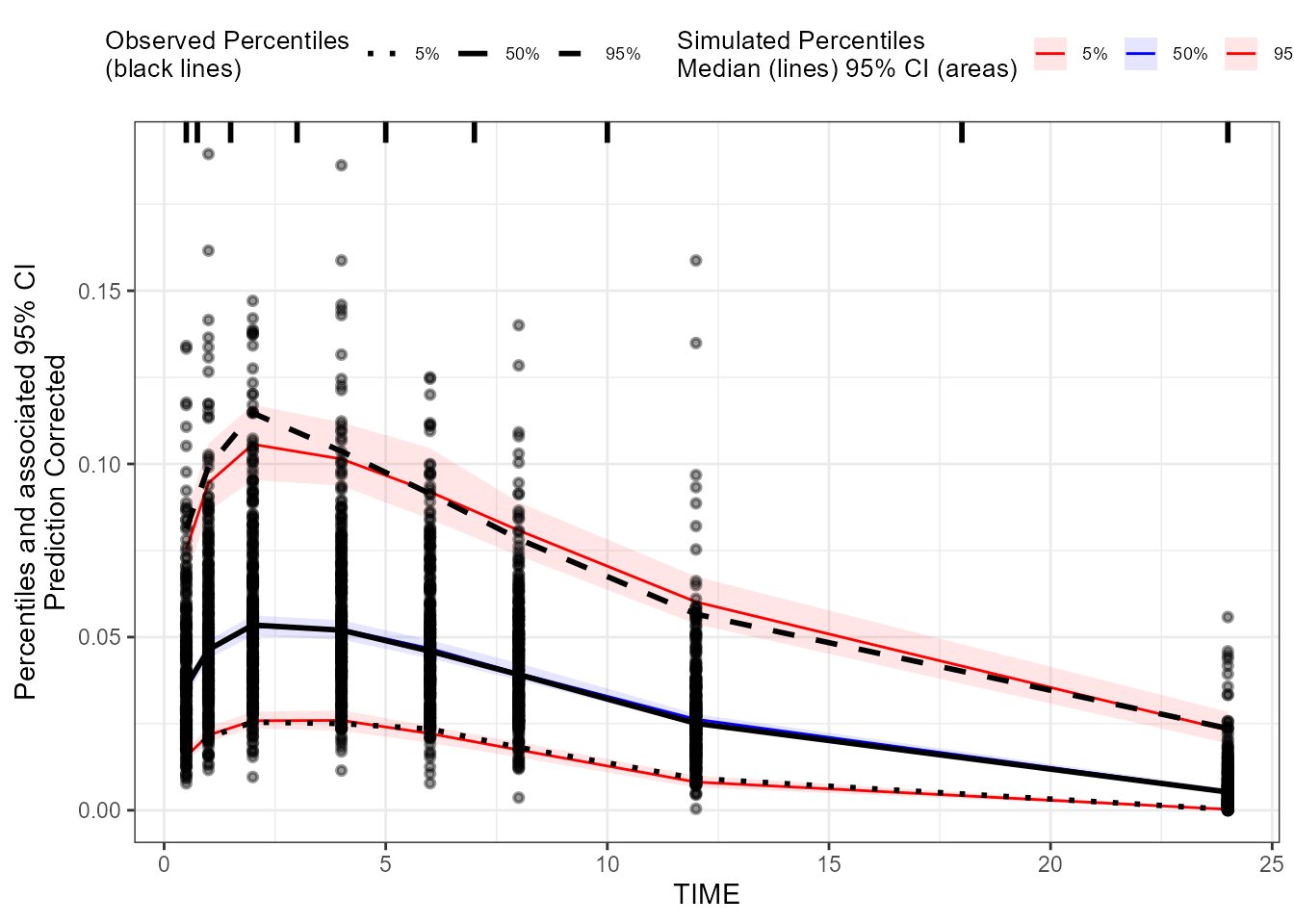### Create a binning VPC plot for CatgoricalObs: binning on x-variable itself
binning_VPC_CategoricalObs <- observed(dt_ObsData_CategoricalObs_tidyvpc,
x = IVAR,
yobs = DV) %>%
simulated(dt_SimData_tidyvpc_CategoricalObs, ysim = DV) %>%
binning(bin = IVAR) %>%
vpcstats(vpc.type = "categorical")

plot(binning_VPC_CategoricalObs
, facet = TRUE
, facet.scales = "fixed"
, legend.position = "bottom"
)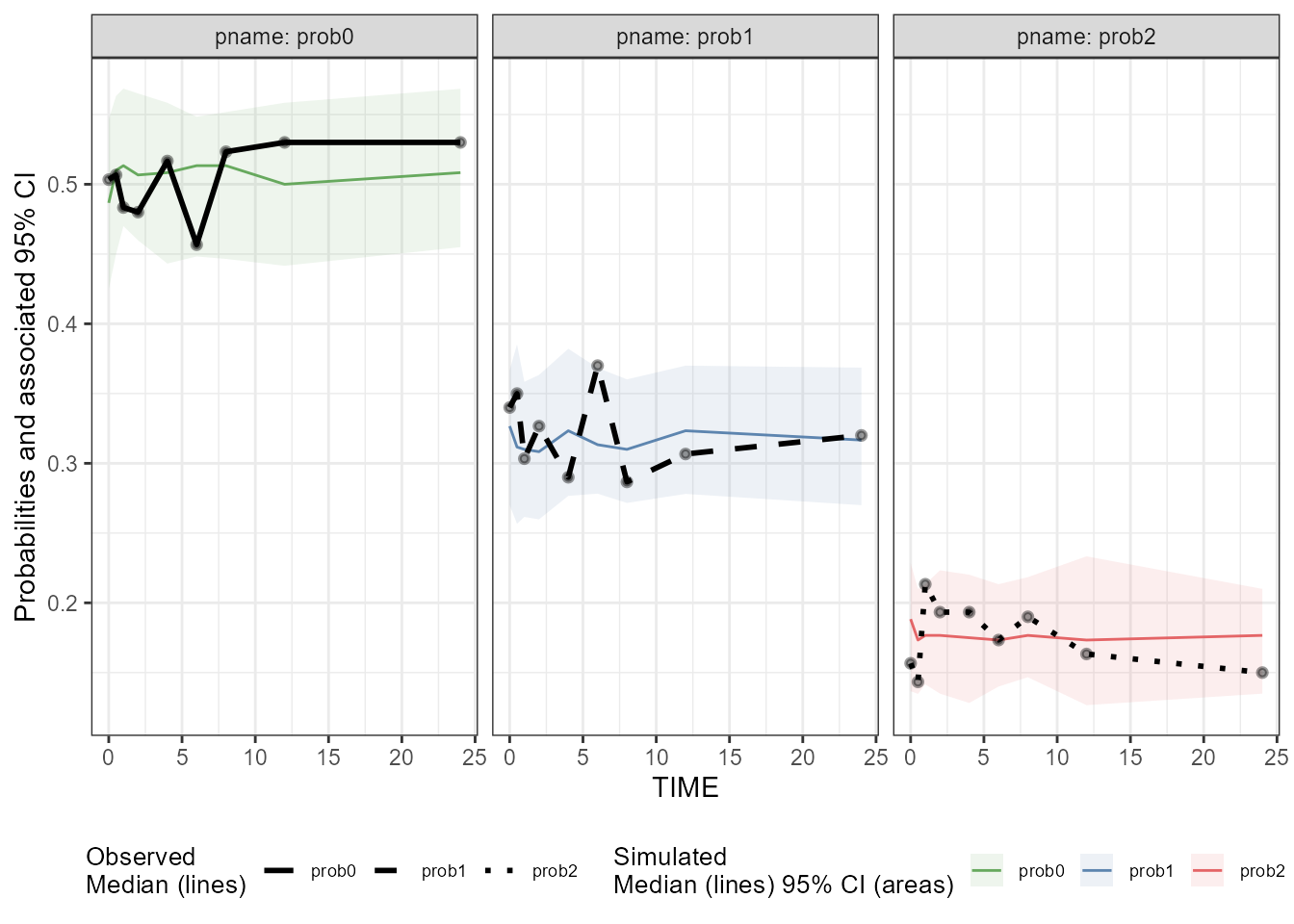Alternatively, one can create/customize VPC plots through VPC results shiny app (in Certara.VPCResults package), which can also be used to:

• generate corresponding tidyvpc code to reproduce the VPC ouput from R command line
• generate report as well as the associated R markdown.

Here we only demonstrate how to invoke this shiny app (Note: The shiny app will automatically preprocess the data as what we did above for tidyvpc package).

library(Certara.VPCResults)
## Invoke VPC results shiny app to create VPC plots for CObs
taggedVPC_CObs <- vpcResultsUI(observed = dt_ObsData_ContinuousObs_tidyvpc_CObs,
simulated = dt_SimData_tidyvpc_CObs)

## Invoke VPC results shiny app to create VPC plots for EObs
taggedVPC_EObs <- vpcResultsUI(observed = dt_ObsData_ContinuousObs_tidyvpc_EObs,
simulated = dt_SimData_tidyvpc_EObs)

## Invoke VPC results shiny app to create  VPC plot for categoricalObs
taggedVPC_CategoricalObs <- vpcResultsUI(observed = dt_ObsData_CategoricalObs_tidyvpc,
simulated = dt_SimData_tidyvpc_CategoricalObs,
vpc.type = "categorical")